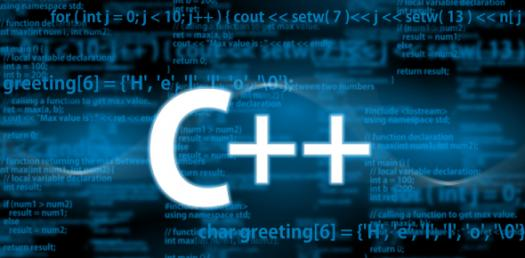# C Plus Plus - From 101 To 222 Multiple Choice

122 Questions | Attempts: 630
ShareSettingsAre you an aspiring programmer and have just started learning C++ language? The quiz below is perfect for you and is from 101 to 222 and is a multiple-choice quiz to help you understand it better. All the best and keep an eye out for some more C++ quizzes. All the best.

• 1.
101. Programs written in an assembly language require a(n) ____, which also is a program, to convert the assembly
• A.

Compiler

• B.

Assembler

• C.

Interpreter

• D.

• 2.
102. The repetition structure is also referred to as a loop or as ____.
• A.

Branch

• B.

Iteration

• C.

Select

• D.

Repeat

• 3.
103. Some high-level languages use a(n) ____ rather than a(n) ____.
• A.

Interpreter, compiler

• B.

Assembler, interpreter

• C.

Assembler, compiler

• D.

• 4.
104. The Windows environment typically uses ____ such as check boxes, list boxes, and buttons.
• A.

Registers

• B.

Objects

• C.

Procedures

• D.

Mnemonics

• 5.
105. The translation of an algorithm into a language that the computer can understand is referred to as ____ the algorithm.
• A.

Paraphrasing

• B.

Coding

• C.

Implementing

• D.

Converting

• 6.
106. A coded algorithm is called a ____.
• A.

Pseudocode

• B.

Library

• C.

Program

• D.

Diagram

• 7.
107. A programmer ____ a program by running it on the computer, using sample data to test the program’s accuracy.
• A.

Evaluates

• B.

Implements

• C.

Benchmarks

• D.

Codes

• 8.
108. The purpose of analyzing a problem is to determine the goal of solving the problem and the items that are needed to
• A.

Output

• B.

Input

• C.

Data

• D.

Start symbol

• 9.
109. Some programmers use a(n) ____ to organize and summarize the results of a problem analysis.
• A.

Flowchart

• B.

IPO chart

• C.

Diagram

• D.

Graph

• 10.
110. In a flowchart, the oval symbol is called the ____ symbol.
• A.

Input/output

• B.

Process

• C.

Selection

• D.

Start/stop

• 11.
111. In a flowchart, the parallelogram symbol is called the ____ symbol.
• A.

• 12.
112. ____ is called the insertion operator.
• A.

• B.

->

• C.

• D.

>>

• 13.
113. ____ is called the extraction operator.
• A.

• B.

->

• C.

• D.

>>

• 14.
114. ____ refers to the process of locating and removing any errors in a program.
• A.

Debugging

• B.

Testing

• C.

• D.

Compiling

• 15.
115. ____ is an example of a syntax error.
• A.

Cout

• B.

Cin >> raiseRate;

• C.

Cout

• D.

Average = number1 + number2 / 2;

• 16.
116. When you compile a Microsoft Visual C++ 2005 program, the compiler generates the appropriate object code, saving it automatically in a file whose filename extension is ____.
• A.

.obj

• B.

.o

• C.

.exe

• D.

.cpp

• 17.
117. A #include ____ provides a convenient way to merge the source code from one file with the source code in another file, without having to retype the code.
• A.

• 18.
118. Variables and ____ are locations (within the computer’s internal memory) where a program can temporarily store data while the program is running.
• A.

Classes

• B.

Literal constants

• C.

Processing items

• D.

Named constants

• 19.
119. Examples of ____ in the C++ programming language include int, double, and return.
• A.

Keywords

• B.

Directives

• C.

Statements

• D.

Identifiers

• 20.
120. ____ is a character literal constant.
• A.

X

• B.

"x"

• C.

‘x’

• D.

\$x

• 21.
121. ____ is a string literal constant.
• A.

X

• B.

""

• C.

‘Hello’

• D.

\$Hello

• 22.
122. When a program instructs the computer to assign a value to a memory location, if the value’s data type does not match the memory location’s data type, the computer uses a process called ____ to convert the value to fit the memory location.
• A.

Implicit type conversion

• B.

Explicit type conversion

• C.

Casting d. translating

• 23.
123. After executing the following lines of code, the result will be ____.int average = 0;double test1 = 90.0;double test2 = 81.0;average = (test1 + test2) / 2;
• A.

85 assigned to average

• B.

85.5 assigned to average

• C.

6 assigned to average

• D.

A syntax error

• 24.
124. The ____ statement tells the computer to convert the double number 3.1 to float, and then assign the result to a float variable named price.
• A.

Float price = 3.1;

• B.

Float price = static_cast3.1;

• C.

Float price = static_castfloat;

• D.

Float price = float3.1;

• 25.
125. The statement ____ creates a string variable named company and initializes it to the empty string.
• A.

String company = '';

• B.

String company = ' ';

• C.

String company = "";

• D.

String company = " ";

## Related TopicsBack to top
×

Wait!
Here's an interesting quiz for you.